# 5 Steps to Calculate Molar Mass of Aluminum

By: Tasha Kolesnikova

7 min

0

10.04.2022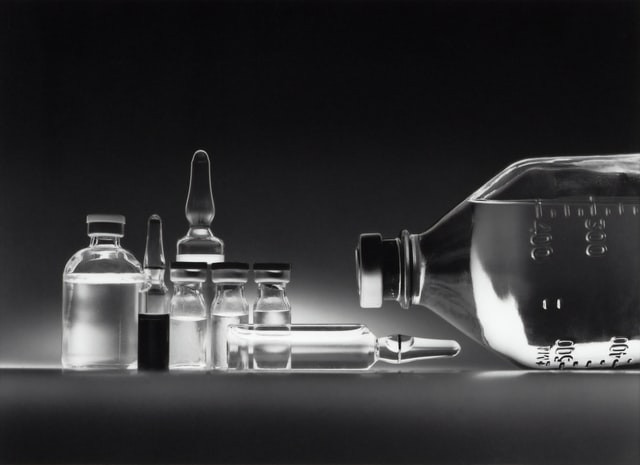Aluminum has found wide application in various fields. The properties of aluminum alloys make them suitable for use in almost all existing industries. This substance is encountered by students when performing chemistry homework or laboratory work. Therefore, we have created a helpful instruction on determining the molar mass of Al since this is the most common task.

## Molar Mass of Aluminum - What Is This Chemical Element?

Name: Aluminum (Al).

Atomic weight: 26,98.

Molecular mass: 26,981539 amu.

Number of atoms: Aluminum contains only one atom, which consists of a positively charged nucleus with 13 protons, 14 neutrons inside, and three electron shells with 13 electrons.

Melting point: 660 °C.

Boiling point: 2518,82 °C.

Molar volume: 10,0 cm³/mol.

Aluminum is a light and malleable white metal, matte silver, thanks to a thin oxide film that immediately covers it in the air. It belongs to the III group of the periodic system, denoted by the symbol Al, has an atomic number of 13 and an atomic mass of 26,98154.

In the 16th century, the famous Paracelsus took the first step towards the extraction of aluminum. From alum, he isolated alum earth, which contained an oxide of a then-unknown metal. In the 18th century, the German chemist Andreas Marggraf returned to this experiment. He named aluminum oxide "alumina," which in Latin means "astringent." At that time, the metal was not popular since people did not find it in its pure form. Only in 1855 in Paris at the World Exhibition, the metal aluminum made a splash.

## How to Find the Molar Mass of Aluminum?

To find the molar mass of aluminum, you need to follow the algorithm:

1. Prepare a periodic table to find out the valence of a given element and atomic mass units.
2. Now you need to write a chemical equation. There is only one element - Al, so the formula will accordingly contain only an aluminum atom in our task. If you had an alumina compound, the equation would be Al2O3. You can make such a formula by learning the valences of the elements in the periodic table.
3. Go back to the periodic table to write down the valence of aluminum. Aluminum in its compounds exhibits a valency equal to III. You will need this data in further calculations.
4. Knowing the valence, you can find the number of moles in aluminum. In our task, there is only one atom, and this is Al, so that the formula will look like this:

M = Ar, Ar (Al) = Al · 1 mol = 26,98 g/mol.

If your substance, for example, would be aluminum sulfate, then its chemical formula looks like this Al2 SO4)3. As you can see, aluminum sulfate contains three elements. Therefore, you need to add up the masses of these elements and find the mole of a substance.
5. You are on your way to the final - there is only one step left. You need to calculate the mass of one mole into aluminum. You can use this formula:

m (Al) = Ðr (Al) / NA = 27 / 6,02 · 1023 = 4,3 · 1023g.

As you can see, we have rounded the number 26,98 to 27 for the convenience of calculations.

If you do not fully understand the algorithm or find it difficult to learn all these formulas, you can always use the help of our paper writing service.

### An Example of How to Use the Molar Mass of Aluminum in a Task

You need to know and find the molar mass of aluminum when solving various problems. As homework help, we'll take a look at one of them.

Decision: On the one hand, the mass of aluminum oxide is: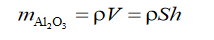In this formula:

• V is the volume of dissolved aluminum oxide;
• ρ is its density;
• h is the thickness of the barrier layer;
• S is the area of the oxide film.

On the other hand, the mass of aluminum in solution is: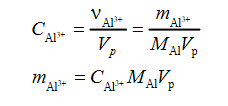In this formula:

• vAl3 + is the amount of aluminum;
• cAl3 + is the concentration of aluminum ionic compounds;
• mAl is the molar mass of aluminum;
• Vp is the volume of the solution;
• mAl3 + is the mass of aluminum in the solution.

Since in aluminum oxide, there are three oxygen atoms per two aluminum atoms, the mass of the oxide can be expressed through the mass of the metal: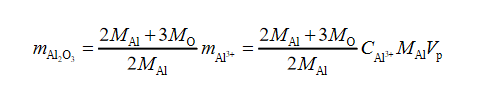Thus, we get the following data: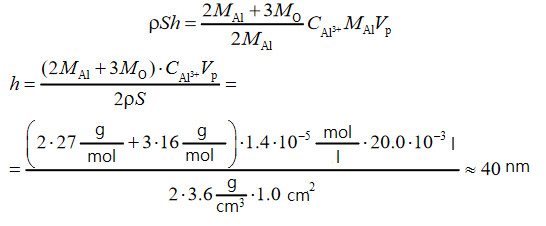From the proposed list, phosphoric and hydrochloric acids can be used to dissolve aluminum oxide: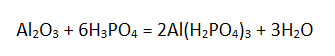It is the acidic salt that is formed since the acid is taken in large excess. Otherwise, the insoluble aluminum phosphate AlPO4 would prevent dissolution.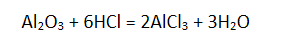Silicic acid cannot be used to dissolve aluminum oxide since it is itself insoluble in water.

## Physical Properties of Aluminum

Aluminum metal is characterized by high electrical conductivity, thermal conductivity, resistance to corrosion and frost, ductility. It lends itself well to stamping, forging, drawing, rolling. Aluminum can be welded well by various types of welding.

Aluminum has a high affinity for oxygen and is covered in the air by a thin, durable aluminum oxide film. This film protects the metal from subsequent oxidation and provides good anti-corrosion properties. Aluminum is resistant to sea, and freshwater practically does not interact with organic acids, concentrated or diluted nitric acid.

## Chemical Properties of Aluminum

Aluminum is a fairly active amphoteric metal. Under normal conditions, a strong oxide film determines its resistance. If the oxide film is destroyed, aluminum acts as a dynamic, reducing metal. At high temperatures, the metal interacts with oxygen. When heated, reactions take place with sulfur, phosphorus, nitrogen, carbon, iodine. Under normal conditions, the metal creates an excellent chemical compound with chlorine, bromine, magnesium, potassium. There is no reaction with hydrogen.

Under the condition of purification from the oxide film, vigorous interaction with water occurs. Reactions with dilute acids proceed quickly. Reactions with concentrated nitric and sulfuric acid occur when heated. Aluminum reacts readily with alkalis. Practical application in metallurgy has found the ability to reduce metals from oxides and salts - the reaction of aluminothermy. Also, as a result of the reaction of aluminum (Al), water (H2O) and sodium chloride (NaCl), aluminum chloride (AlCl3), hydrogen (H2), sodium hydroxide (NaOH) are formed.

## Isotopes of Aluminum

It is known that in nature, aluminum can be in the form of one stable isotope 27Al. The mass number is 27. The nucleus of the 27Al aluminum isotope contains thirteen protons and fourteen neutrons. There are radioactive isotopes of aluminum with mass numbers from 21 to 42, among which the most long-lived isotope 26Al, which has a half-life of 720 thousand years.

## How Do People Get Aluminum?

Aluminum is among metals and third among all elements in terms of abundance in the earth's crust. This reagent constitutes approximately 8% of the earth's crust, and aluminum is found in the tissues of animals, plants as a trace element. In nature, it occurs in a bound form in the form of rocks, minerals. The stone shell of the earth, which is at the base of the continents, is formed precisely by aluminosilicates, silicates.

Most often, people get aluminum from bauxite. Deposits of this mineral are common in countries of the tropical and subtropical zones. When extracting aluminum from nepheline, people also get potash, soda ash, cement, and fertilizers along the way.

## Where Is Aluminum Used?

People use aluminum in metallurgy as a base for alloys and an alloying element. People use aluminum alloys in everyday life, architecture and construction, shipbuilding and automotive, space and aviation technology. Chemists use aluminum in the production of explosives. Companies use anodized aluminum to make jewelry. Aluminum nitrate is used in textile mills to etch fabrics before dyeing, plays a vital role in leather tanning, and is used to make filaments.

User ratings:

User ratings is 4.3 stars.